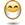# Formula for cell differences

## Excel Help for Formula For Cell Differences in Analyzing Corporate Financial DataIn cell one I enter an amount this amount may change. Cell two is multiplied by cell three. The answer is in cell four. The answer in cell four can not be larger than cell one. If it is smaller that number can appear. If lager than cell one I would like the difference to be in cell five. Posted by marcel on 23 Nov 2010Rate this: (3/5 from 1 vote) Lets suppose cells one to three are in range A1:A3.  The formula for cell A4 is:=IF((A2*A3)>A1,"",(A2*A3))The formaula for cell A5 is then:=IF(A4="",(A2*A3)-A1,"") Excel Business Forums Administrator Posted by Excel Helper on 23 Nov 2010